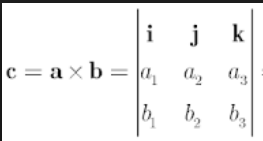Q

# Solve! - Vector Algebra - JEE Main-4

Let

and a vector    be such that

and then   equals

• Option 1)

11/3

• Option 2)

• Option 3)

• Option 4)

92 Views

As we have learned

Vector Product of two vectors -- wherein

Properties of Scalar Product -

-

thus

and

also

y=2/3 and z=  2/3  and x= 5/3

Option 1)

11/3

This is incorrect

Option 2)

This is incorrect

Option 3)

This is correct

Option 4)

This is incorrect

Exams
Articles
Questions